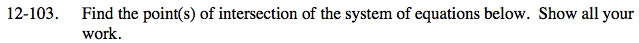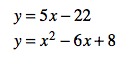### Home > CA > Chapter Ch12 > Lesson 12.4.4 > Problem12-103

12-103.

Find the point(s) of intersection of the system of equations below. Show all your work. Homework Help ✎

y = 5x − 22
y = x2 − 6x + 8The graph of these equations would be a line and a parabola.
How many possible intersection points are there?

Use the Equal Values method to solve.

Set the new equation equal to 0 and then solve for x by factoring.

Remember to find the y-value that corresponds to each x-value.